Courses

# Practice Questions with Solutions: HCF & LCM Notes | EduRev

## Class 5 : Practice Questions with Solutions: HCF & LCM Notes | EduRev

The document Practice Questions with Solutions: HCF & LCM Notes | EduRev is a part of the Class 5 Course Mathematics for Class 5.
All you need of Class 5 at this link: Class 5

Question 1: Find the H.C.F. of 15 and 28.

15 = 1 × 15, 15 = 3 × 5.

All the factors of 15 are 1, 3, 5 and 15.

Also, 28 = 28 × 1, 28 = 2 × 14, 28 = 4 × 7

All the factors of 28 are 1, 2, 4, 7, 14 and 28.

Common factor of 15 and 28 is 1.

Thus, H.C.F. of 15 and 28 is 1.

We call 15 and 28 are co-prime.

Question 2: Find all the common factors of :

(a) 24 = 1 × 24

24 = 2 × 12,

24 = 3 × 8, 24 = 4 × 6

Therefore, all the factors of 24 are 1, 2, 3, 4, 6,

8, 12 and 24.

Also, 40 = 1 × 40, 40 = 2 × 20, 40 = 4 × 10,

40 = 5 × 8.

Therefore, all the factors of 40 are 1, 2, 4, 5, 8, 10, 20 and 40.

Thus, common factors of 24 and 40 are 1, 2, 4 and 8.

(b) 20 and 64

20 = 1 × 20, 20 = 2 × 10, 20 = 4 × 5

Therefore, all the factors of 20 are 1, 2, 4, 5, 10 and 20.

Also, 64 = 1 × 64, 64 = 2 × 32, 64 = 4 × 16, 64 = 8 × 8.

Therefore, all the factors of 64 are 1, 2, 4, 8, 16, 32 and 64.

Thus, common factors of 20 and 64 are 1, 2 and 4.

Question 3: Find the H.C.F. of 18, 45 and 63.

18 = 1 × 18, 18 = 2 × 9, 18 = 3 × 6

All the factors of 18 are 1, 2, 3, 6, 9 and 18.

Also, 45 = 1 × 45, 45 = 3 × 15, 45 = 5 × 9.

All the factors of 45 are 1, 3, 5, 9, 15 and 45.

Also, 63 = 1 × 63, 63 = 3 × 21, 63 = 7 × 9.

All the factors of 63 are 1, 3, 7, 9, 21 and 63.

Common factors of 18, 45 and 63 are 1, 3 and 9.

Thus, H.C.F. of 18, 45 and 63 is 9.

Question 4: Find the L.C.M. of 12 and 18.

Multiples of 12 are 12, 24, 36, 48, 60, 72, .....

Multiples of 18 are 18, 36, 54, 72, 90, .....

Thus, common multiples of 12 and 18 are 36, 72, .....

Therefore, L.C.M. of 12 and 18 is 36.

Question 5: Find the first two common multiples of :

(a) 4 and 10

Multiples of 4 are 4, 8, 12, 16, 20, 24, 28, 32, 36, 40, .....

Multiples of 10 are 10, 20, 30, 40, .....

Thus, the first two common multiples of 4 and 10 are 20 and 40.

(b) 5, 6 and 15.

Multiples of 5 are 5, 10, 15, 20, 25, 30, 35, 40, 45, 60, 65, .....

Multiples of 6 are 6, 12, 18, 24, 30, 36, 42, 48, 54, 60, .....

Multiples of 15 are 15, 30, 45, 60, 75, .....

Thus, the first two common multiples of 5, 6 and 15 are 30 and 60.

Question 6: Write in the product form :

(a) 87

87 = 8 × 8 × 8 × 8 × 8 × 8 × 8

(b) 63 × 114

63 × 114 = 6 × 6 × 6 × 11 × 11 × 11 × 11.

Question 7: Write in the exponential form :

(a) 5 × 5 × 5 × 5 × 5 × 5

5 × 5 × 5 × 5 × 5 × 5 = 56

(b) 8 × 8 × 8 × 8 × 9 × 9

8 × 8 × 8 × 8 × 9 × 9 = 84 × 92.

Question 8: Draw a factor tree to find the prime factorisation of 96.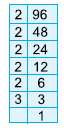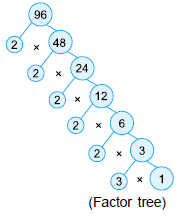Thus, the prime factorisation of 96 is

= 2 × 2 × 2 × 2 × 2 × 3 = 25 × 3.

Question 9: Express 56 as the product of prime factors.

We proceed as follows :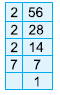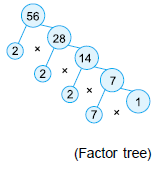Divide 56 by 2

Divide 28 by 2

Divide 14 by 2

Divide 7 by 7

Hence, the prime factorisation of 56 is

= 2 × 2 × 2 × 7 = 23 × 7.

Question 10: Find the H.C.F. of 14, 84 and 105.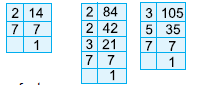Resolving the given numbers into prime factors, we get.

14 = 2 × 7

84 = 2 × 2 × 3 × 7 = 22 × 3 × 7

105 = 3 × 5 × 7

H.C.F. of 14, 84 and 105 = Product of common prime factors

= Product of terms with smallest powers of common factors.

= 71 = 7

Thus, the H.C.F. of 14, 84 and 105 = 7.

Question 11: Find the H.C.F. of 28 and 72 by prime factorisation method.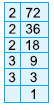Resolving each of the given numbers into prime factors, we get

28 = 2 × 2 × 7 = 22 × 7

72 = 2 × 2 × 2 × 3 × 3 = 23 × 32

H.C.F. of 28 and 72 = Product of common prime factors

= Product of terms with smallest powers of common factors

= 22

= 4

Thus, H.C.F. of 28 and 72 = 4.

Question 12: Find the H.C.F. of 144, 180 and 324.

We first find the HCF of any two numbers (144 and 180).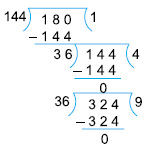Since, last divisor is 36, so 36 is the HCF of 144 and 180.

Now, we find the HCF of 36 and 324.

Hence, HCF of 144, 180 and 324 is 36.

Question 13: Find the L.C.M. of 16, 48 and 64.

Resolving each of the given numbers into prime factors, we get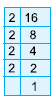16 = 2 × 2 × 2 × 2 = 24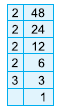48 = 2 × 2 × 2 × 2 × 3 = = 2× 3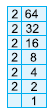64 = 2 × 2 × 2 × 2 × 2 × 2 = 26

The different prime factors of 16, 48 and 64 are 2 and 3.

Thus, L.C.M. of 16, 48 and 64 = Product of terms containing highest powers of 2 and 3.

= 26 × 3

= 64 × 3 = 192.

Question 14: Find the L.C.M. of 15 and 40, using prime factorisation method.

Resolving the given numbers into prime factors, we get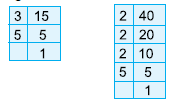So, 15 = 3 × 5

Also, 40 = 2 × 2 × 2 × 5 = 23 × 5.

The different prime factors of 15 and 40 are 2, 3 and 5.

L.C.M. of 15 and 40 = Product of terms containing highest powers of 2, 3 and 5

= 2× 3 × 5 = 8 × 3 × 5 = 120.

Question 15: Find the LCM of 18, 36 and 42 by long division method.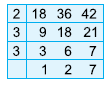So, LCM of 18, 36 and 42

= 2 × 3 × 3 × 2 × 7 = 252

Hence, LCM of 18, 36 and 42 = 252.

Question 16: The product of two numbers is 867 and their HCF is 17. Find their L.C.M.

We know that:

LCM =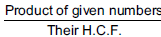= 867/17 = 51

Hence, the LCM of given numbers is 51.

Question 17: The H.C.F. of two numbers is 52 and their L.C.M is 312. If one of the numbers is 104, find the other.

It is given here that:

H.C.F. = 52, L.C.M. = 312 and one number = 104

The other number =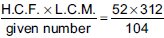= 156

Hence, the other number is 156.

Offer running on EduRev: Apply code STAYHOME200 to get INR 200 off on our premium plan EduRev Infinity!

## Mathematics for Class 5

26 videos|48 docs|35 tests

,

,

,

,

,

,

,

,

,

,

,

,

,

,

,

,

,

,

,

,

,

;